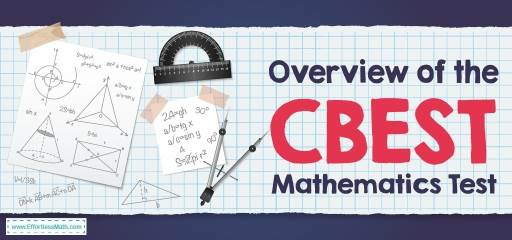# Overview of the CBEST Mathematics TestThe California Basic Educational Skills Test, or CBEST, is a test required for people who would like to teach in public schools in the state of California.

The CBEST has three sections:

• Writing
• Mathematics

There are 50 multiple-choice questions in each math and reading section, and the writing section requires two essay responses. Test-takers have four hours to complete the exam, and there is no time limit for each section. Depending on your local test site, you may take a computer-based exam or a paper-and-pencil test.

## What are the topics in CBEST Mathematics?

The math section of the CBEST test not only assesses your ability to perform various math functions and answer problems but also knows how to use math knowledge in an assortment of commonplace teaching situations. Many of the questions you will find in this test are only related to the concepts you learned in high school. The mathematics section is as follows:

### Estimation, measurement, and statistics (30%):

This section deals with your ability to evaluate statistical properties and functions, as well as estimating numerical and measurement-based data. This section is further divided into two separate subsets:

#### Statistical Principles:

The questions involve percentiles, probability, and averages, among various other basic elements of statistics. You should also demonstrate your knowledge of standardized tests and how they are scored. Some questions provide hypothetical grade information that you should use to compare a student’s grades with the rest of the group.

#### Estimation and Measurement:

This section evaluates your numerical guessing abilities. The questions of the following subset require you to figure out the ballpark answer for an arithmetic equation. Find measurements in specific conditions or contexts (such as capacity or temperature). Analyze a time-sensitive situation through the word problem and infer how long it takes to do a particular task, and measure the perimeter and various other elements of the geometric shape.

### Computation and problem solving (35%):

The questions will require you to use your knowledge of basic arithmetic functions—division, subtraction, multiplication, and addition. You are also expected to analyze word problems and find answers to them through a technique different from the norm. Also, find out if you can come up with a reasonable answer to a problem based on the data provided.

### Number and graph relationships (35%):

This section focuses primarily on data – charts, graphs, spreadsheets, tables, and percentages. You must use the data provided to answer the questions. Discover how two pieces of data relate to one another. Fill in the blanks of the data sheets based on the patterns in the other inputs. And understand the different rules of data.

## Is the CBEST mathematics hard?

CBEST math is hard because this test is designed to ensure the high skill of the prospective teacher. Therefore, it is important to be prepared.

## Do you get a formula sheet in theCBEST mathematics?

You will not be given a formula in the CBEST math test, so you will need to memorize all the formulas required for the test.

## Can you use a calculator on the CBEST mathematics?

The CBEST mathematics subtest does not allow any candidate to use any type of calculator. This means that everyone who takes the CBEST math subtest must do the calculations themselves.

## What is the passing score of CBEST mathematics?

You must score at least 41 to pass the math subtest.

Looking for the best resource to help you succeed on the CBEST Math test?

## More from Effortless Math for CBEST Test …

### Are you looking for the best score-raising study guides?

5 Best CBEST Math Study Guides article helps you choose the best.

### Are you looking for a new approach to learning CBEST math?

The Ultimate CBEST Math Course (+ FREE Worksheets & Tests) will pave the way for you to learn.

### Does thinking about exam day make you anxious?

Do not worry, you will succeed by following the tips of the exam day: CBEST Math-Test Day Tips

## Have any questions about the CBEST Test?

### What people say about "Overview of the CBEST Mathematics Test - Effortless Math: We Help Students Learn to LOVE Mathematics"?

No one replied yet.

X
49% OFF

Limited time only!

Save Over 49%

SAVE \$34

It was \$69.99 now it is \$35.99